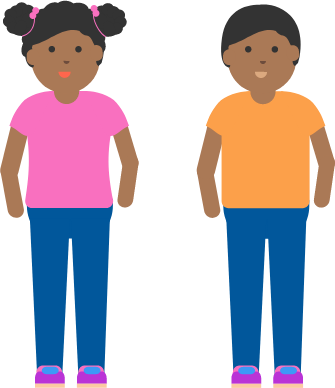### Probability Fundamentals

Suppose you roll a fair, $6$-sided die three times. Which sequence of rolls is most likely?# Common Fallacies

You have flipped a fair coin $9$ times and it has landed on tails all $9$ times in a row. What is the probability that the next flip will be tails?# Common Fallacies

Every week you play a lottery game that requires choosing $4$ numbers between $1$ and $80.$ In this particular game, you win "outright" and do not have to potentially share the prize with others. Your friends give you some advice for the next week on which numbers to pick.

Which advice is the most reasonable?

A. You should choose two even and two odd numbers.

B. You should not pick $4$ consecutive numbers $($numbers in a row such as $3, 4, 5, 6).$

C. You should pick some low numbers, high numbers, and numbers in the middle.

D. It doesn't matter which four numbers you pick. It's just luck.

# Common Fallacies

Which is more likely?A. You roll one die $6$ times and the rolls follow the ordered pattern $123456.$

B. You roll $6$ dice all at once, and you’re able to arrange them to make the unordered pattern $123456.$

# Common Fallacies

Assuming that a set of parents is not trying to have children at any particular time of the year, what is the probability that, in a family with two children, the two children share the same birthday?×